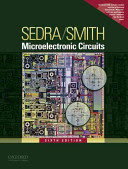### Electronics 1

#### Learning Outcomes

1. explain the structure and operation principle of basic semiconductor devices
2. explain the electrical charcteristics of basic semiconductor devices
3. analyze basic electronic amplifiers
4. compare the amplifiers with different electronic devices
5. analyze CMOS logic circuits
6. analyze of electronic circuits using electronic computers
7. apply basic measurements in electronic laboratory

#### Forms of Teaching

Lectures

Lectures

Exercises

Problem solving

Laboratory

Lab excercises

Continuous Assessment Exam
Laboratory Exercises 0 % 12 % 0 % 12 %
Mid Term Exam: Written 0 % 44 % 0 %
Final Exam: Written 0 % 44 %
Exam: Written 0 % 50 %
Exam: Oral 38 %
##### Comment:

Before final exam students must have completed all laboratory exercises.

#### Week by Week Schedule

1. Application and importance of electronics; Introduction to amplifiers (gain, input and output resistance).
2. Introduction to amplifiers (gain, input and output resistance); Introduction to digital electronics.
3. Intrinsic and extrinsic semiconductors; Carrier concentrations, electrons and holes.
4. Carrier concentrations, electrons and holes; Current flow in semiconductors (drift and diffusion current).
5. Operating principle; Electrical characteristics.
6. Small-signal model; Optoelectronic electron devices; Basic rectifier circuits.
7. Structure and operating principle of MOSFET and JFET; Electrical characteristics; Small-signal model; Definition and stabilization of bias operating point.
8. Midterm exam.
9. Basic amplifier configurations: common source, common gate, common drain; Calculation of gain, input and output resistances of basic amplifier configurations; Calculation of output voltage swing.
10. CMOS inverter; Combinational CMOS circuits; Sequential CMOS circuits.
11. Structure and operating principle of BJT; Electrical characteristics; Small-signal model.
12. Definition and stabilization of bias operating point; Basic amplifier configurations: common emitter, common base, common collector; Calculation of gain, input and output resistances of basic amplifier configurations; Calculation of output voltage swing.
13. Basic amplifier configurations: common emitter, common base, common collector; Calculation of gain, input and output resistances of basic amplifier configurations.
14. Inverters with bipolar transistors; Current switch with BJT and basic ECL circuit.
15. Final exam.

#### Study Programmes

Computing (study)
Elective course from a group of mandatory courses-Electronics 1 (3. semester)
Electrical Engineering and Information Technology (study)
(3. semester)

#### Literature

Ž. Butković, J. Divković-Pukšec, A. Barić (2018.), Elektronika 1, Fakutet elektrotehnike i računarstva, Zagreb - interna skripta, Fakutet elektrotehnike i računarstva Sveučilišta u ZagrebuA.S. Sedra, K.C. Smith (2011.), Microelectronic Circuits, 6th ed., Oxford University Press
R.C. Jaeger, T.N. Blalock (2011.), Microelectronic Circuit Design, 4th ed., McGraw-Hill

#### General

ID 183399
Winter semester
6 ECTS
L3 English Level
L1 e-Learning
60 Lectures
15 Exercises
12 Laboratory exercises
0 Project laboratory